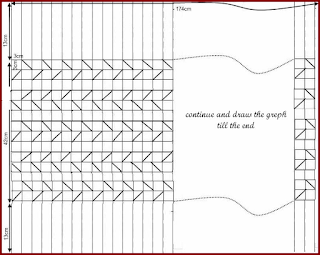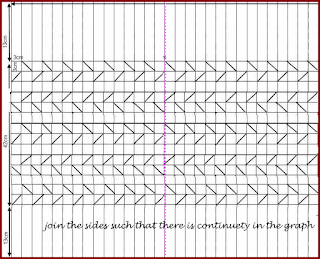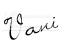## Wednesday, December 8, 2010

### Method of calculating material need for Canadian smocking for "Matrix design"This design contains repetition of 2 columns of squares marked with diagonals (shown in pink colour) and one column which is left blank (Shown in green colour). Finally ends with 2 columns of squares marked with diagonals.Tthat means there should be odd numbers of columns marked with diagonals  separated by Gaps. In other words first and last columns should be columns marked with diagonals. Numbers of repetitions of these three columns depend upon the size of the cushion and the size of the square of the graph used.
Smocking is done on pairs of columns marked with diagonals together. After completing one pair of column we have to move on to the next pair.

Width of the material after smockingAfter smocking the width of one pair of column of squares become equal to ½ if the diagonal of the square used.he gap takes the shape of an equilateral triangle (See the diagram 1) whose side is equal to the diagonal of the square. Width of the gap will be equal to the height of the triangle thus formed.
Height of the triangle can be calculated by using the diagonal of the square used
Height of the triangle =√ (diagonal²–½diagonal²) .No need to worry about the formula. I have given the table which gives the diagonal and height of the triangle formed when smocking is done on different sizes of squares.Length

After smocking the height of two rows will be equal to the diagonal of the square
With the help of these calculations we can find out the number of squares we need in each row and in each column. And then the material needed..Figures3,4and 5 explain the changes that take place after smocking for different sizes of squaresI will give one example
How to calculate material needed for a cushion of size 40cm x40cm smocking done on graph with squares 3cm x 3cm
Width
After smocking width first column becomes half of the diagonal =2.1cm
Width of next gap will be equal to height of the triangle formed = 3.6cm
Total of these three squares will be 5.7cm
These three squares are repeated
Last column is column of squares with diagonals (marked pink) its width is 2.1cm
Subtract 2.1 cm from 40 cm =37.9 cm
Now we have to find out how many times the fist 3 squares whose width will be 5.7 can be repeated to get to get 37.9cm
37.9cm÷ 5.7= 6.6
We can repeat 6 times without fraction that means we have to repeat 3 squares 6 times
3x6= 18 squares + last column 2 squares. = 20 squares
If you smock on 20 squares resulting material will be (8x 5.7) + 2.1= 34.2 cm + 2.1cm= 36.3 cm
It is 37  cm approximately  or 4cm less than 40 cm this can be made up by adding 2 cm on either side as border to this add 1cm on either side for seams
Length

We get 4, 2 cm when we smock 2 rows
These two rows should be repeated 40 ÷ 4.2= 9.5 times or 9 times without taking decimal
2 rows X 9 = 18 rows. When we smock these 18 rows the length we get = 4.2 x 9= 37.8 cm
It is 2.2 cm less than 40 cm. it can be made up by adding 1 .1 cm on either side. To this add 1 cm on either side as border.
So the graph will have 18 rows each row having 20 squares
Width of the material=20 squares x 3cm=60cm + 6cm=66cm
Length of the material = 18 X 3 cm =54 cm + 4.2 cm=56.2 cm
Material needed =66 cm Χ 56.2 cm## Sunday, December 5, 2010

### Method of calculating material need for Canadian smocking -"Arrow head design"

Material need for Canadian smocking depends upon the flowing things

1. Size of the finished material we need.
2. Design we select.
3. Size of the square in the graph we use for smocking.

For each design I have arrived at a general formula using mathematics .By just substituting the numbers in the formula we can find out  the width of the material by calculating how many squares we need in a row. We can calculate the length of the material needed by calculating how many squares we need in a column.
Diagonal of the square plays a great role in this. I will give you approximate values diagonals of the squares we normally use.
Diagonal of a square of 2 cm =2 .8 cm
Diagonal will be for square of 2 .5 cm = 3.5 cm
Diagonal will be for square of 3 cm = 4.2 cm
Diagonal will be for square of 4 cm= 5.7 cm

Method of calculating material need for a cushion of "Arrow head design"Cushion size 40 cm X 40 cmSize of the square used in the graph= 3 cm

General formula to calculate width of the material

No of squares needed in row = (width of the finished smocked material needed x 4) ÷ diagonal of the square
General formula to calculate length of the material

No of squares in a column = (length of the finished smocked material needed X 2) ÷ Diagonal of the square

Method of calculating the width of the material

No of squares needed in a row = (40 cm X 4) ÷ 4.2 = 38 squares
Material needed for 38 squares =38 X 3 cm = 114 cm
Width of the material needed =114 cm + 2 cm  for seams =116 cm

Method of calculating the length of the material

No of squares in a column = (length of the finished smocked material needed X 2) ÷ Diagonal of the square
No of squares in a column = (40 cm X 2) ÷ 4.2 = 19 squares
Material needed for 19 squares =19 X 3 cm =57 cm + 2 cm for seams =59 cms.Please note that the number of squares in a row should be of even number.
First row and last row are smocked by joining 2 points

The remaining rows are smocked in pairs by the method of joining 3 points.
In the same manner we can calculate the material needed  for different sizes of  cushions done with different sizes of squares.

## Friday, December 3, 2010

### "Flower basket" in dimensional embroidery

In this design I have used stitches which are used in Brazilian embroidery. I have used double cast on stitch for small pink flowers which are wrapped by daisy stitch.It has got an embossed effect.For big flowers I have used pistil stitches of different lengths.Flower centers are done with French knots.I did applique work for basket ,once again I have used double cast on stitch for the bars.## Thursday, October 7, 2010

### White cushions with Canadian smocking reverse of 'Bee cottage design"

These cushions are done by  the method " Joining 4 points. " It is the reverse of " Bee cottage " design.Smocking is done on graph of 3cm squares.Border of 7.5cm is marked  all around.Finished smocked piece will beof size( 7.5cm +3cm+9cm+3cm+9cm+3cm+7.5cm) =42cm x 42cm. Size of finished cushion will be 40cm x 40cm

## Tuesday, August 31, 2010

### The method of making round cushion with Canadian smocking part 1graph of round cushionJoin the sidesMaterials needed

Satin cloth 70 cm x 176 cm
2 big cloth covered buttons

I could not get buttons of the size I wanted. I got plastic disks having 5 cm diameter cut from school badge maker, made two holes using hot nails, passed a thick thread through the holes and tied a knot. I then covered them with sponge sheet and fabric.

Design used -- leaf design
Size of the square used --3cm x 3cm

Mark the graph as shown in the figure leaving 1 cm all around for seams. Squares should come in the center and the parallel lines on the sides. You can do the smocking by just drawing the squares in the middle.I prefer to draw lines at the sides also. We can use these lines as guide lines while pleating. Finish the edges by Zigzag stitches. This stops the edges from fraying and hindering our work while smocking. The squares that are marked along the length (176 cm) should always be of an even number. This decides the circumference of the cushion. The squares marked along the width depend upon the design and thickness of the cushion we want. The length of the parallel line decides the diameter of the cushion.

Join the shorter edges together matching the graph as shown in the figure to form a tube. Do the smocking by joining two points together. This forms a cover for a round cushion

Measure the circumference and width of the smocked tube. Stitch inner cushion of the same size of the smocked piece as illustrated in the figures.
Cover the inner cushion with smocked cover as shown in the figures.

I have used the smocked piece to make a cushion cover and not to make a cushion. By doing so we can smock as many covers as we want and can store them in a small place.We can change them whenever we want by untying the knot that holds the the pleats on one side. Long thread can be used while stitching the pleats and then the extra thread remains after tying cab be pushed in side the opening.By doing so we need not stitch the pleats again. Washing covers is easier than washing cushions.
There is another method in which poly fill is directly filled in the smocked cover and openings are covered with cloth covered buttons.

Continued in newer posts.............